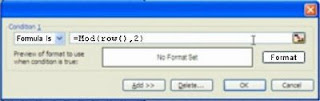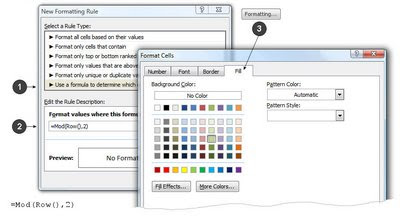## Sunday, June 14, 2009

### Excel: Coloring Alternate Rows

Excel How To Automatically Color Alternating Rows in Excel using Conditional Formatting. How to highlight every 5th row. (Keywords: Greenbar; every other row)

Follow these steps to "conditionally" format cells so they have alternating colors on every other row (or other number). Doing this can make a sheet easier to read and follow. The formatting automatically adjusts when new rows and columns are inserted or deleted.Steps for Excel 2003 and Older
(Steps for Excel 2007, below)

1. Highlight the cell range where you want the alternating rows or highlight the entire rows.

3. In the 'Condition 1' pull-down, select "Formula Is"• In the Formula bar, type this formula, including the equal-sign:
=Mod(Row(),2)
• Click the Format Button.
• Along the top row of tabs, choose [Patterns]. See below for an illustration.
• Change the Background color to a light tan or soft-green
• Okay, Okay

Steps for Excel 2007(with the Ribbon Bar Menu; see above for older versions of Excel)

1. Highlight the range of cells where you want the alternating rows to be applied or select the entire rows.

2. On the "Home" Ribbon, select "Conditional Formatting".
Click image for a larger view; click Back to return.3. Select New Rule
• In the Rule Type Dialog, choose "Use a formula to determine which cells to format"
• In the formula bar type this formula:
=Mod(Row(),2)
• If copying and pasting, be sure you don't end up with any quotes, as in ="Mod(Row(), 2)"
• Click the "Format..." button; then choose the [Fill] tab.
• Choose your favorite color; click OK, OKVariations - Every 5th row:

The formula:  =Mod(Row(),5) highlights all rows except those divisible by 5 -- in other words, each row, except those evenly divisible by 5, would get a "1,2,3, or 4" in the remainder.  This inverts the highlights, where every row is highlighted but the fifth ones.  This is probably not what you want.

To highlight every 5th row, use a slightly more complicated formula that resolves to a True or False:

=IF(MOD(ROW(),5)<>0,FALSE,TRUE)

Change the word "Row" to "Column" to highlight alternating columns.

The "row()" function returns the current row-number. As an aside, =row(C15) returns row-15.

The Mod function divides the current row number by (2) and records the "remainder" (remember grade-school division?). With this division, the rows are either odd or even (a remainder of zero or one).

Notice the Conditional format is set to trigger when the formula is "true". Here is the trick: In Excel-lingo, a "1" is true and a zero (0) is false (actually, in this case, any number greater than zero is considered True). Because the MOD formula always resolves to a 1 or zero (T/F), this is enough to set the conditional and trigger the formatting. A simplified example:Notice how the row you highlighted can be either shaded or not -- the calculation is based on the physical row number, not on where you started the process.

Performance Considerations:

Applying a conditional format to individual cells introduces extra calculations with each cell.

If applied to tens of thousands of cells, this has the potential to impact performance. Although I have not tested this, I suspect conditional formatting applied to the Rows (rather than the cells) is more efficient because the rows would inherit a default formatting and each cell within would not have to be re-calculated.

On large sheets, I recommend applying conditional formatting to the rows instead of individual cells.Modifying Existing Rules:
To change an already-built rule, click "Conditional Formatting", Manage Rules. Select the specific rule to change; then "Edit Rules".

Click "Conditional Formatting", "Clear Rules" to remove the rule.

Related Keyliner Articles:
Excel UDF (User Defined Functions)
Using Excel for Raffle-Ticket Drawing: Prizeorama
Excel VLookup - a complete tutorial
Excel Coloring Alternate Rows
Excel Parsing City-State-Zip
Excel Importing Text with Leading Zeroes
VB - Return First Word, Last Word, Supertrim
Using VBA to Send Email
Using Excel to select Raffle Tickets - Prize-orama